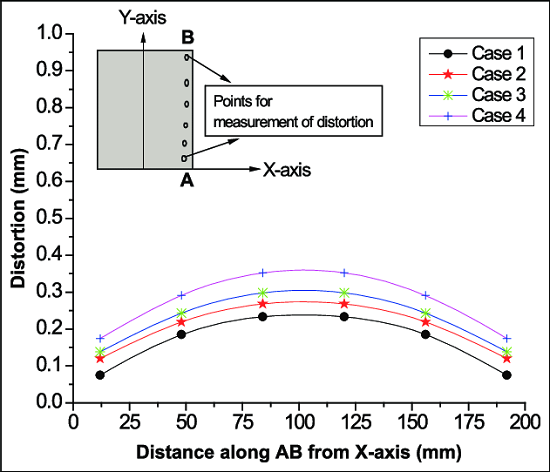# How to avoid the distortion that occurs in the transmission line?

What are the types of line distortions?

The distortions occurring in the transmission line are called waveform distortion or line distortion. Waveform distortion is of two types i.e. frequency distortion and phase or delay distortion.

How frequency distortion occurs in a power transmission line?

When a signal having many frequency components are transmitted along the line, all the frequencies will not have equal attenuation and hence the received end waveform will not be identical with the input waveform at the sending end because each frequency is having different attenuation. This type of distortion is called frequency distortion.

In order to reduce frequency distortion occurring in the line,

• The attenuation constant α should be made independent of frequency.
• By using equalizers at the line terminals which minimize the frequency distortion. Equalizers are networks whose frequency and phase characteristics are adjusted to be inverse to those of the lines, which result in a uniform frequency response over the desired frequency band, and hence the attenuation is equal for all the frequencies.

### What is delay distortion?

When a signal having many frequency components are transmitted along the line, all the frequencies will not have same time of transmission, some frequencies being delayed more than others. So the received end waveform will not be identical with the input waveform at the sending end because some frequency components will be delayed more than those of other frequencies. This type of distortion is called phase or delay distortion.### How to avoid the frequency distortion that occurs in the line?

In order to reduce frequency distortion occurring in the line,

a) The phase constant β should be made dependent of frequency.
b) The velocity of propagation is independent of frequency.
c) By using equalizers at the line terminals which minimize the frequency distortion. Equalizers are networks whose frequency and phase characteristics are adjusted to be inverse to those of the lines, which result in a uniform frequency response over the desired frequency band, and hence the phase is equal for all the frequencies.

What is a distortion less line? What is the condition for a distortion less line?

A line, which has neither frequency distortion nor phase distortion is called a distortion less line. The condition for a distortion less line is RC=LG.  Also,

• The attenuation constant α should be made independent of frequency.
• The phase constant β should be made dependent of frequency.
• The velocity of propagation is independent of frequency.

#### What is the drawback of using ordinary telephone cables?

In ordinary telephone cables, the wires are insulated with paper and twisted in pairs, therefore there will not be flux linkage between the wires, which results in negligible inductance, and conductance. If this is the case, the there occurs frequency and phase distortion in the line.

How the telephone line can be made a distortion less line?

For the telephone cable to be distortion less line, the inductance value should be increased by placing lumped inductors along the line.